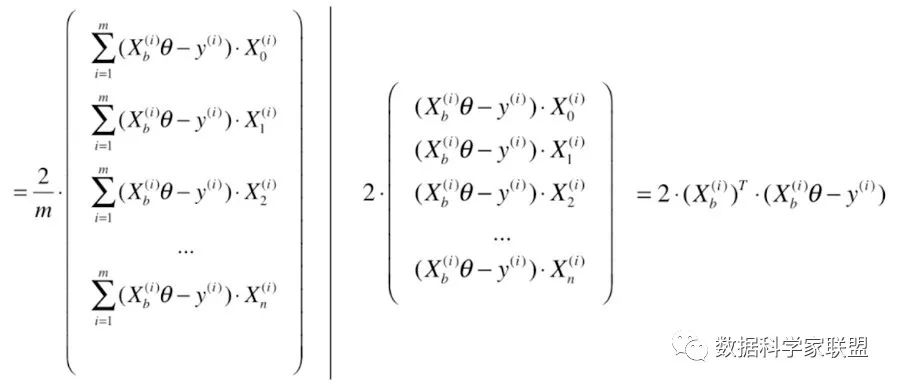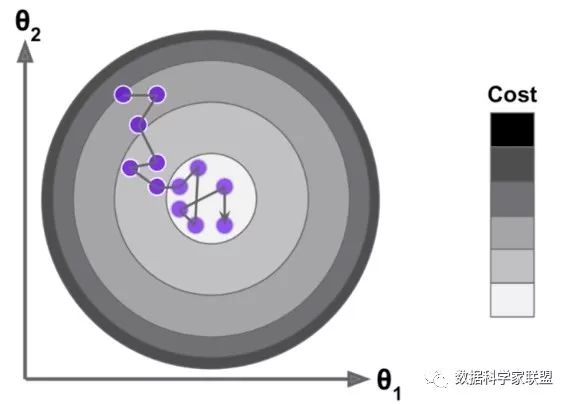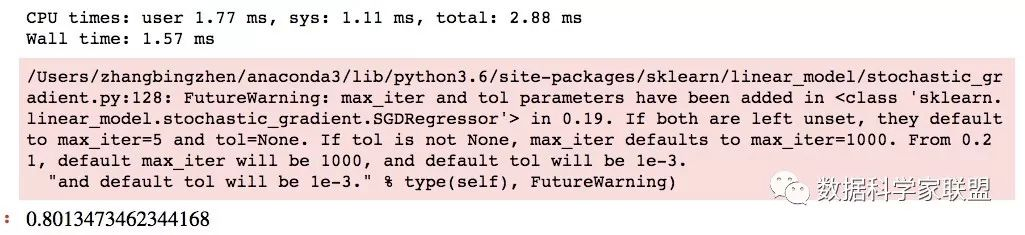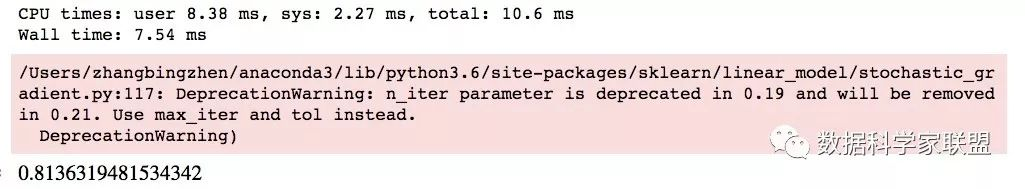• 随机梯度下降 随机梯度下降是一种用于解决使用凸损失函数的线性分类器的方法，如，支持向量机和逻辑回归。
随机梯度下降
随机梯度下降是一种用于解决使用凸损失函数的线性分类器的方法，如，支持向量机和逻辑回归。它在大规模学习的背景下，吸引了大量的注意。
随机梯度下降的优点：

快速
易实现

随机梯度下降的缺点：

需要大量的超参，如，正则化参数和迭代次数
对特征缩放敏感

分类
在拟合模型时，确保在每次迭代后，打乱训练数据。
SGDClassifier实现了一个基本的随机梯度学习函数，支持不同的损失函数和惩罚。
损失函数包括：

loss='hinge：(soft-margin) linear Support Vector Machine
loss='modified_huber'：smoothed hinge loss
loss='log'：logistic regression
and all regression losses below

惩罚包括：

penalty='l2'：L2范数
penalty='l1'：L1范数
penalty='elasticnet'：L2和L1范数的凸组合；(1 - l1_ratio) * L2 + l1_ratio * L1

SGDClassifier以one versus all (OVA)方式组合多个二元分类器来支持多类别分类。对$K$个类别中的每一类，学习这个类和其它$K-1$个类的差别得到一个二元分类器。在测试时，计算每类距超平面的带符号的距离，选择具有最大距离的类。
# coding: utf-8
# SGD: Maximum margin separating hyperplane

import numpy as np
import matplotlib.pyplot as plt
from sklearn.linear_model import SGDClassifier
from sklearn.datasets import make_blobs

X, Y = make_blobs(n_samples=50, centers=2, random_state=0, cluster_std=0.60)

clf = SGDClassifier(loss='hinge', alpha=0.01, max_iter=2000)
clf.fit(X, Y)

xx = np.linspace(-1, 5, 10)
yy = np.linspace(-1, 5, 10)

X1, X2 = np.meshgrid(xx, yy)
Z = np.empty(X1.shape)
# np.ndenumerate: Return an iterator yielding pairs of array coordinates and values
for (i, j), val in np.ndenumerate(X1):
x1 = val
x2 = X2[i, j]
p = clf.decision_function([[x1, x2]])
Z[i, j] = p

levels = [-1.0, 0.0, 1.0]
linestyles = ['dashed', 'solid', 'dashed']
colors = 'k'
plt.contour(X1, X2, Z, levels, colors=colors, linestyles=linestyles)
plt.scatter(X[:, 0], X[:, 1], c=Y, cmap=plt.cm.Paired, edgecolor='black', s=20)

plt.axis('tight')
plt.show()

回归
类SGDRegressor实现了一种随机梯度下降的函数，支撑不同的惩罚函数和惩罚来拟合线性回归模型。SGDRegressor适用超过10000以上训练样本的回归问题，对于其它问题，推荐使用Ridge、Lasso和ElasticNet。
通过loss参数，可设置的损失函数包括：

loss = 'squared_loss'：Ordinary least squares
loss = 'huber'：Huber loss for robust regresson
loss='epsilon_insensitive'：linear support vector regression

Huber和epsilon-insensitive损失函数能够被用于鲁棒回归。不敏感区域的宽度可通过参数epsilon参数设置。这个参数依赖于目标变量的尺度。
SGDRegressor支持平均的SGD作为SGDClassifier。通过设置参数average=True。
对稀疏数据的随机梯度下降
由于对截距的收缩学习率，稀疏实现得到的结果与密集的稍有不同。一般使用scipy.sparse和scipy.sparse.csr_matrix格式的矩阵。
终止条件
SGDClassifier和SGDRegression提供了两种终止准则：

当early_stopping = True，输入数据被划分为一个训练集和一个验证集，模型拟合到训练集，终止规则基于在验证集上的预测分数。验证集的大小能够按照参数validation_fraction的值变化。
当early_stopping = False，模型拟合在整个数据集上，终止规则基于输入数据上计算的目标函数。

使用提醒

随机梯度下降对特征尺度比较敏感，因此要对数据做缩放。例如，将每个输入数据的属性值缩放到[0, 1]或[-1, +1]，或者是标准化到均值为0，方差为1。注意，同样的缩放需应用到测试集上，已获得有意义的结果。这些可使用	StandardScaler类完成
使用GridSearchCV找到合理的正则化参数alpha
经验上，在观察近10^6个训练样本时，SGD趋于收敛。因此，一个合理的迭代次数设置为max_iter = np.ceil(10**6/n)，n是训练集的大小
如果将SGD应用于使用PCA提取的特征，将特征值缩放一个使训练数据平均l2范数等于1的c值
ASGD在有大量特征和较大值eta0的情况下，表现最好

数学模型
给定一个训练集$(x_1, y_1), ... (x_n, y_n)$，其中，$x_i \in R^m$和$y_i \in {-1, 1}$，目标是学习一个线性打分函数$f(x) = w^Tx + b$，其中，模型参数$w \in R^m$和截距$b \in R$。为了做预测，需看$f(x)$的符号。一个常用的选择是找到模型参数，以最小化正则训练误差：
$E(w, b) = \frac{1}{n}\sum\limits_{i=1}^{n}L(y_i, f(x_i)) + \alpha R(w)$
其中，$L$是一个损失函数和$R$是一个正则项惩罚模型的复杂度；$\alpha > 0$是一个非负的超参。
不同的损失函数对应不同的分类器

Hinge：(soft-margin) Support Vector Machines
Log：Logistic Regression
Least-Squares：Ridge Regression
Epsilon-Insensitive：(soft-margin) Support Vector Regression

常用的正则项如下

L2 范数：$R(w) = \frac{1}{2}\sum\limits_{i=1}^{n}w_{i}^{2}$
L1范数：$R(w) = \sum\limits_{i=1}^{n}|w_i|$，会产生稀疏的解
Elastic Net：$R(w) = \frac{\rho}{2}\sum\limits_{i=1}^{n}w_{i}^{2}+(1-\rho)\sum\limits_{i=1}^{n}|w_i|$，一个L2和L1范数的凸组合。


展开全文机器学习 python
• Contents1 梯度下降和随机梯度下降1.1 一维梯度下降1.2 学习率1.3 多维梯度下降1.4 随机梯度下降2 小批量随机梯度下降2.1 读取数据2.2 从零开始实现2.4 小批量随机梯度下降简洁实现 1 梯度下降和随机梯度下降 1.1 一...
Contents1 梯度下降和随机梯度下降1.1 一维梯度下降1.2 学习率1.3 多维梯度下降1.4 随机梯度下降2 小批量随机梯度下降2.1 读取数据2.2 从零开始实现2.4 小批量随机梯度下降简洁实现
1 梯度下降和随机梯度下降
1.1 一维梯度下降
%matplotlib inline
import numpy as np
import torch
import math
import sys
sys.path.append('..')
import d2lzh_pytorch as d2l

def gd(eta):
x = 10
results = [x]
for i in range(10):
x -= eta * 2 * x
results.append(x)
print('epoch 10, x:',x)
return results
gd(0.2)

epoch 10, x: 0.06046617599999997

def show_trace(res):
n = max(abs(min(res)), abs(max(res)), 10)
f_fine = np.arange(-n, n, 0.1)
d2l.set_figsize()
d2l.plt.plot(f_fine, [x * x for x in f_fine])
d2l.plt.plot(res, [x*x for x in res], '-o')
results=gd(0.2)
show_trace(results)

epoch 10, x: 0.060466175999999971.2 学习率
# 学习率不可以过大，也不可以过小
# 如果使用过小的学习率，会导致x更新缓慢从而需要更多的迭代次数才能得到较好的解。
show_trace(gd(0.05))

epoch 10, x: 3.4867844009999995# 学习率过大会使一阶泰勒展开公式不再成立
show_trace(gd(1.1))

epoch 10, x: 61.9173642240000961.3 多维梯度下降
def train_2d(trainer):
x1 , x2, s1, s2 = -5, -2, 0, 0
results = [(x1, x2)]
for i in range(20):
x1 , x2, s1, s2 = trainer( x1 , x2, s1, s2)
results.append((x1,x2))
print('epoch %d, x1 %f, x2 %f' % (i+1, x1, x2))
return results

def show_train_2d(f, results):
d2l.plt.plot(*zip(*results), '-o', color='#ff7f0e')
x1, x2 = np.meshgrid(np.arange(-5.5,1,0.1), np.arange(-3.0,1.0,0.1))
d2l.plt.contour(x1, x2, f(x1,x2), 8, colors='green')
d2l.plt.contourf(x1, x2, f(x1,x2), 8, alpha=.75, cmap='gray_r')

eta=0.1
def f_2d(x1,x2):
return x1**2 + 2 * x2 **2
def gd_2d(x1, x2, s1, s2):
return x1 - eta * 2*x1, x2-eta*4*x2, 0, 0

show_train_2d(f_2d, train_2d(gd_2d))

epoch 20, x1 -0.057646, x2 -0.0000731.4 随机梯度下降
def sgd_2d(x1, x2, s1, s2):
return (x1-eta*(2*x1+np.random.normal(0,1)),x2-eta*(4*x2+np.random.normal(0,1)), 0, 0)

show_train_2d(f_2d, train_2d(sgd_2d))

epoch 20, x1 -0.044038, x2 -0.1462582 小批量随机梯度下降
2.1 读取数据
%matplotlib inline
import numpy as np
import time
import torch
from torch import nn, optim
import sys
sys.path.append("..")
import d2lzh_pytorch as d2l

def get_data_ch7():
data = np.genfromtxt('E:/Coding/Jupyter_note/data/airfoil_self_noise.dat', delimiter = '\t')
data = (data - data.mean(axis = 0)) / data.std(axis = 0)
return torch.tensor(data[: 1500, : -1], dtype = torch.float32), torch.tensor(data[:1500,-1], dtype = torch.float32)
features, labels =  get_data_ch7()
features.shape

torch.Size([1500, 5])

2.2 从零开始实现
def sgd(params, status, hyperparams):
for p in params:
def trian_ch7(optimizer_fn, states, hyperparams, features , labels, batch_size = 10, num_epochs = 2):
net, loss = d2l.linreg, d2l.squared_loss
w = torch.nn.Parameter(torch.tensor(np.random.normal(0,0.01, size = (features.shape, 1)), dtype = torch.float32), requires_grad=True)
b = torch.nn.Parameter(torch.zeros(1,dtype = torch.float32), requires_grad=True)

def eval_loss():
return loss(net(features, w, b), labels).mean().item()

ls = [eval_loss()]
data_iter = torch.utils.data.DataLoader(torch.utils.data.TensorDataset(features , labels), batch_size, shuffle = True)

for _ in range(num_epochs):
start = time.time()
for batch_i, (X,y) in enumerate(data_iter):
l = loss(net(X, w, b), y).mean()

l.backward()
optimizer_fn([w,b], states, hyperparams)
if(batch_i+1)*batch_size%100==0:
ls.append(eval_loss())
print('loss: %f, %f sec per epoch' % (ls[-1], time.time()-start))
print(len(ls))
d2l.set_figsize()
d2l.plt.plot(np.linspace(0, num_epochs, len(ls)), ls)
d2l.plt.xlabel('epoch')
d2l.plt.ylabel('loss')

# 普通梯度下降
def train_sgd(lr,  batch_size = 10, num_epochs = 2):
trian_ch7(sgd, None, {'lr':lr}, features, labels, batch_size, num_epochs)

train_sgd(1, 1500, 6)

loss: 0.245595, 0.020942 sec per epoch
7# 当批量大小为1时，优化使用的是随机梯度下降。
train_sgd(0.005, 1)

loss: 0.246122, 0.536564 sec per epochtrain_sgd(0.05,10)

loss: 0.248938, 0.090757 sec per epoch2.4 小批量随机梯度下降简洁实现
def train_pytroch_ch7(optimizer_fn, optimizer_hyperparams, features , labels, batch_size = 10, num_epochs = 2):

net = nn.Sequential(
nn.Linear(features.shape[-1], 1)
)
loss = nn.MSELoss()

optimizer = optimizer_fn(net.parameters(), **optimizer_hyperparams)

def eval_loss():
return loss(net(features).view(-1), labels).item() / 2

ls = [eval_loss()]
data_iter = torch.utils.data.DataLoader(torch.utils.data.TensorDataset(features , labels), batch_size, shuffle = True)

for _ in range(num_epochs):
start = time.time()
for batch_i, (X, y) in enumerate(data_iter):
l = loss(net(X).view(-1), y) / 2

l.backward()
optimizer.step()
if(batch_i+1)*batch_size % 100 == 0:
ls.append(eval_loss())

print('loss: %f, %f sec per epoch' % (ls[-1], time.time()-start))
print(len(ls))
d2l.set_figsize()
d2l.plt.plot(np.linspace(0, num_epochs, len(ls)), ls)
d2l.plt.xlabel('epoch')
d2l.plt.ylabel('loss')


train_pytroch_ch7(optim.SGD, {'lr': 0.05}, features , labels, batch_size = 10)

loss: 0.244927, 0.081793 sec per epoch参考原文：《动手学深度学习（pyTorch）》
欢迎关注【OAOA】展开全文python 深度学习 神经网络
梯度下降（gradient descent）：遍历全部数据集算一次损失函数，然后算函数对各个参数的梯度，更新梯度。这种方法每更新一次参数都要把数据集里的所有样本都看一遍，需要计算所有的样本然后求平均，其计算得到的是一个标准梯度。计算量开销大，计算速度慢，不支持在线学习，这称为Batch gradient descent，批梯度下降。
批处理的随机梯度下降（Min-batch SGD）：用一些小样本来近似全部的，其本质就是既然1个样本的近似不一定准，那就用更大的30个或50个样本来近似。将样本分成m个mini-batch，每个mini-batch包含n个样本；在每个mini-batch里计算每个样本的梯度，然后在这个mini-batch里求和取平均作为最终的梯度来更新参数，即：每跑一个batch就更新一次参数；然后再用下一个mini-batch来计算梯度，如此循环下去直到m个mini-batch操作完就称为一个epoch结束。==目前对于深度神经网络的训练，均采用批处理的随机梯度下降算法（mini-batch SGD）


展开全文• 0x00 前言 在之前介绍的梯度下降法的步骤中，在每次更新参数时是需要计算所有样本的，通过对整个数据集的所有...针对该缺点，有一种更好的方法：随机梯度下降法SGD(stochastic gradient descent)，随机梯度下降是...


0x00 前言 在之前介绍的梯度下降法的步骤中，在每次更新参数时是需要计算所有样本的，通过对整个数据集的所有样本的计算来求解梯度的方向。这种计算方法被称为：批量梯度下降法BGD(Batch Gradient Descent)。但是这种方法在数据量很大时需要计算很久。针对该缺点，有一种更好的方法：随机梯度下降法SGD(stochastic gradient descent)，随机梯度下降是每次迭代使用一个样本来对参数进行更新。虽然不是每次迭代得到的损失函数都向着全局最优方向，但是大的整体的方向是向全局最优解的，最终的结果往往是在全局最优解附近。但是相比于批量梯度，这样的方法更快，我们也是可以接受的。下面就来学学随机梯度下降法吧！0x01 理解随机梯度下降 1.1 随机取值的公式推导批量梯度下降法，每一次计算过程，都要将样本中所有信息进行批量计算。但是显然如果样本量很大的话，计算梯度就比较耗时。基于这个问题，改进的方案就是随机梯度下降法。即每次迭代随机选取一个样本来对参数进行更新。使得训练速度加快。下面我们从数学的角度来看：我们将原本的矩阵中根据样本数量求和这一操作去掉，同样也就不需要除以m了。最终我们得到损失函数的进行求导，得到的结果为：要注意，得到的向量是搜索方向，不是梯度方向，因此已经不是算是函数的梯度了。1.2 随机下降与学习率的取值其过程就是：每次随机取出一个i，得到一个向量，沿着这个随机产生的向量的方向进行搜索，不停的迭代，得到的损失函数的最小值。随机梯度下降法的搜索过程如下图所示。如果是批量搜索，那么每次都是沿着一个方向前进，但是随机梯度下降法由于不能保证随机选择的方向是损失函数减小的方向，更不能保证一定是减小速度最快的方向，所以搜索路径就会呈现下图的态势。即随机梯度下降有着不可预知性。但实验结论告诉我们，通过随机梯度下降法，依然能够达到最小值的附近(用精度换速度)。随机梯度下降法的过程中，学习率的取值很重要，这是因为如果学习率一直取一个固定值，所以可能会导致点已经取到最小值附近了，但是固定的步长导致点的取值又跳去了这个点的范围。因此我们希望在随机梯度下降法中，学习率是逐渐递减的。设计一个函数，使学习率随着下降循环次数的增加而减小。我们想到最简单的表示方法是一个倒数的形式，不过这样也会有问题，如果循环次数比较小的时候，学习率下降的太快了，比如循环次数为1变为2，则学习率减少50%。因此我们可以将分子变为常数，并在分母上增加一个常数项来来缓解初始情况下，学习率变化太大的情况，且更灵活。0x02 BGD&SGD效果比较 下面可以我们要对比一下，批量梯度下降算法和随机梯度下降算法的比较。我们主要观察运行时间和迭代次数这两个指标。2.1 批量梯度下降法代码import numpy as npimport matplotlib.pyplot as pltm = 100000x = np.random.normal(size=m)X = x.reshape(-1,1)y = 4. * x + 3. + np.random.normal(0, 3, size=m)def J(theta, X_b, y):    try:        return np.sum((y-X_b.dot(theta)) ** 2) / len(y)    except:        return float('inf')def dJ(theta, X_b, y):    return X_b.T.dot(X_b.dot(theta) - y) * 2. / len(y)def gradient_descent(X_b, y, initial_theta, eta, n_iters=1e4, epsilon=1e-6):    theta = initial_theta    cur_iter = 0    while(cur_iter < n_iters):        gradient = dJ(theta, X_b, y)        last_theta = theta        theta = theta - eta * gradient        if (abs(J(theta, X_b, y) - J(last_theta, X_b, y)) < epsilon):            break        cur_iter += 1    return theta%%timeX_b = np.hstack([np.ones((len(X),1)),X])initial_theta = np.zeros(X_b.shape)eta = 0.01theta = gradient_descent(X_b, y, initial_theta, eta)theta"""CPU times: user 2.3 s, sys: 493 ms, total: 2.79 sWall time: 1.73 sarray([3.00328612, 4.00425536])"""2.2 随机梯度下降首先，计算损失函数的求导结果时，传递的不是整个矩阵和整个向量，而是其中一行X_b_i、其中的一个数值，因此表达式为X_b_i.T.dot(X_b_i.dot(theta) - y_i) * 2.然后我们可以内置一个计算学习率的函数，即使用来缓解初始情况下，学习率变化太大的情况。最后就是终止循环条件的差异了。在批量梯度下降的计算过程中，循环终止的条件有两个，第一个是循环次数达到上限；第二个是找到的损失函数减小的值不能在减小预设精度这么多了。对于批量梯度下降来说，循环终止的条件有两个，第一个是循环次数达到上限，第二个是找到的损失函数减小的值不能在减小预设精度这么多了那么在随机梯度下降中，由于梯度下降的方向是随机的，所以损失函数不能保证一直减小，有可能是跳跃的，因此就不要第二个条件了，直接使用for循环判断迭代次数就好了。# 传递的不是整个矩阵X_b，而是其中一行X_b_i;传递y其中的一个数值y_idef dJ_sgd(theta, X_b_i, y_i):    return X_b_i.T.dot(X_b_i.dot(theta) - y_i) * 2.def sgd(X_b, y, initial_theta, n_iters):    t0 = 5    t1 = 50    #     def learning_rate(cur_iter):        return t0 / (cur_iter + t1)    theta = initial_theta    for cur_iter in range(n_iters):        # 随机找到一个样本(得到其索引)        rand_i = np.random.randint(len(X_b))        gradient = dJ_sgd(theta, X_b[rand_i], y[rand_i])        theta = theta - learning_rate(cur_iter) * gradient    return theta%%timeX_b = np.hstack([np.ones((len(X),1)),X])initial_theta = np.zeros(X_b.shape)theta = sgd(X_b, y, initial_theta, n_iters=len(X_b)//3)print(theta)"""输出：[2.9287233  4.05019715]CPU times: user 296 ms, sys: 6.49 ms, total: 302 msWall time: 300 ms"""2.3 分析通过简单的例子，我们可以看出，在批量梯度下降的过程中消耗时间1.73 s，而在随机梯度下降中，只使用300 ms。并且随机梯度下降中只考虑的三分一的样本量，且得到的结果一定达到了局部最小值的范围内。0x03 代码实现 3.1 改进后的代码在上一小节，为了比较批量梯度下降和随机梯度下降，我们进行了简单的实现，但实际上对于随机梯度下降法还是有一些问题的。在之前，只是简单的只考虑的三分一的样本量，实际上我们已经考虑所有的样本量n次，然后在每次考虑样本量时采用随机的方式。我们首先要了解：表示对所有样本的循环次数，所有样本的个数为m。因此我们在循环时，应该使用双重循环，即外层循环为每次循环所有样本，内层循环为在所有样本中进行随机选择，次数为样本数量。那么要注意：在计算学习率时，次数就变为def fit_sgd(self, X_train, y_train, n_iters=50, t0=5, t1=50):        """根据训练数据集X_train, y_train, 使用梯度下降法训练Linear Regression模型"""        assert X_train.shape == y_train.shape, \            "the size of X_train must be equal to the size of y_train"        assert n_iters >= 1        def dJ_sgd(theta, X_b_i, y_i):            return X_b_i * (X_b_i.dot(theta) - y_i) * 2.        def sgd(X_b, y, initial_theta, n_iters=5, t0=5, t1=50):            def learning_rate(t):                return t0 / (t + t1)            theta = initial_theta            m = len(X_b)            for i_iter in range(n_iters):                # 将原本的数据随机打乱，然后再按顺序取值就相当于随机取值                indexes = np.random.permutation(m)                X_b_new = X_b[indexes,:]                y_new = y[indexes]                for i in range(m):                    gradient = dJ_sgd(theta, X_b_new[i], y_new[i])                    theta = theta - learning_rate(i_iter * m + i) * gradient            return theta        X_b = np.hstack([np.ones((len(X_train), 1)), X_train])        initial_theta = np.random.randn(X_b.shape)        self._theta = sgd(X_b, y_train, initial_theta, n_iters, t0, t1)        self.intercept_ = self._theta        self.coef_ = self._theta[1:]        return self3.2 使用自己的SGDimport numpy as npfrom sklearn import datasetsboston = datasets.load_boston()X = boston.datay = boston.targetX = X[y < 50.0]y = y[y < 50.0]from myAlgorithm.LinearRegression import LinearRegressionfrom myAlgorithm.model_selection import train_test_splitX_train, X_test, y_train, y_test = train_test_split(X, y, seed=666)standardScaler = StandardScaler()standardScaler.fit(X_train)X_train_std = standardScaler.transform(X_train)X_test_std = standardScaler.transform(X_test)lin_reg1 = LinearRegression()lin_reg1.fit_sgd(X_train, y_train, n_iters=2)lin_reg1.score(X_test_std, y_test)"""输出：0.78651716204682975"""通过增加的值，可以获得更好的结果：lin_reg1.fit_sgd(X_train, y_train, n_iters=100)lin_reg1.score(X_test_std, y_test)"""输出：0.81294846132723497"""3.3 sklearn中的SGDfrom sklearn.linear_model import SGDRegressorsgd_reg = SGDRegressor()    # 默认n_iter=5%time sgd_reg.fit(X_train_std, y_train)sgd_reg.score(X_test_std, y_test)速度非常快！增加迭代次数，可以提升效果sgd_reg = SGDRegressor(n_iter=100)%time sgd_reg.fit(X_train_std, y_train)sgd_reg.score(X_test_std, y_test)sklearn中的算法实现，其实和我们的实现有很大的区别，进行了很多的优化。我们只是简单的实现了它的原理，感兴趣的同学可以去康康相关源代码。0xFF总结 批量梯度下降法BGD(Batch Gradient Descent)。优点：全局最优解；易于并行实现；缺点：当样本数据很多时，计算量开销大，计算速度慢。针对于上述缺点，其实有一种更好的方法：随机梯度下降法SGD(stochastic gradient descent)，随机梯度下降是每次迭代使用一个样本来对参数进行更新。优点：计算速度快；缺点：收敛性能不好

展开全文机器学习 深度学习
• 0 前言 在之前介绍的梯度下降法的步骤中，在每次更新参数时是需要计算所有样本的，通过对整个...针对该缺点，有一种更好的方法：随机梯度下降法SGD（stochastic gradient descent），随机梯度下降是每次迭代使用一个...
• 相反，随机梯度下降,每一步在训练集中随机选择一个实例，并且仅基于该单个实例来计算梯度。 与使用批量梯度下降相比，随机梯度下降时，每个训练步骤要快得多，但也更加随机 当成本函数非常不规则时，随机梯度...算法 python 深度学习 机器学习 人工智能
• 原文 原创 梯度下降与随机梯度下降概念及推导过程 置顶 ...
• 随机梯度下降（SGD）：每次迭代随机使用一组样本 针对BGD算法训练速度过慢的缺点，提出了SGD算法，普通的BGD算法是每次迭代把所有样本都过一遍，每训练一组样本就把梯度更新一次。而SGD算法是从样本中随机抽出一组...
• 1，梯度下降方法的缺点。...2，随机梯度下降是对梯度下降的一个优化。 1）用部分样本的损失代表总体的损失，减少损失计算时间。 3，随机梯度下降的缺点 1）部分样本不能充分代表总体（在少部分数据中） ...
• 在梯度下降的三种类型(Batch梯度下降、Mini-batch梯度下降和随机梯度下降)中，我应该使用哪一种呢?在这篇文章中，我们将了解这些概念之间的区别，并从梯度下降的代码实现来阐明这些方法。梯度下降梯度下降是帮助神经...逻辑回归 深度学习 机器学习 python 人工智能
• 对比梯度下降和随机梯度下降和批量梯度下降 之前看的知识比较零散，没有一个系统的解释说明，看了一些网上的博主的分析，总结了一下自己的理解。 梯度下降：梯度下降就是我上面的推导，要留意，在梯度下降中，......